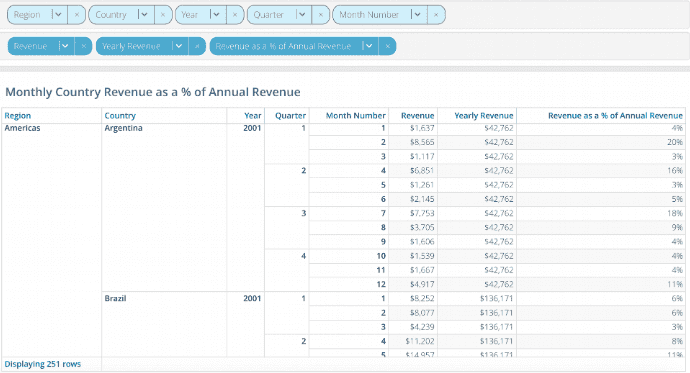Aggregation Functions

Aggregation functions are used to summarize the data in a column. Applying them returns a single value. For example, min returns the smallest value in a column.

Functions Operating on Numeric Scalars in a Column

The functions in this table operate on numeric scalars. The only allowed type is double.

Function Syntax Description
average() average(double[] exp) Compute the average in a column
median() median(double[] exp) Find the median in a column
min() min(double[] exp) Find the minimum value in a column
max() max(double[] exp) Find the maximum value in a column
sum() sum(double[] exp) Find the sum of the values in a column
sum() sum(double[] exp, groupBy) Find the sum of values for each group

Functions Counting Items of Any Type in a Column

The functions in this table operate on any Incorta data type. For this table, ”<item>” stands for any Incorta data type.

Function Syntax Description
count() count(<item>) Returns the number of elements in a column
distinct() distinct(<item>) Returns the number of distinct groups of elements in a column

Aggregation with Level Based Measure

Aggregation functions can take, as a second argument, the groupBy parameter. This is an optional parameter and is valuable when creating a Level based measure.

In the example below, the user wants to create an Insight to show the percentage contribution of monthly revenue to annual revenue by country and region.To do this, the user needs to create two derived formula columns:

1. Yearly Revenue, a measure aggregated at a higher level than the monthly grain and repeated for each of the monthly grain data values.
2. Revenue at the monthly grain/Yearly Revenue Alan: not sure if I got bullet number 2 correctNote the sequence of the groupBy dimensions in the formula is important and should match the sequence of grouping dimensions in the insight. In the insight above you will see Region, Country and then Year in the grouping dimension tray.